# 为什么我要放弃javaScript数据结构与算法（第十章）—— 排序和搜索算法

## 第十章 排序和搜索算法

### 排序算法

function ArrayList(){
var array = [];
this.insert = function(item){
array.push(item);
}
this.toString = function(){
return array.join();
}
}


ArrayList 是一个简单的数据结构，它将项存储在数组。我们只需要一个插入方法来向数据结构中添加元素。使用js原生的push方法即可，而改写toString函数运用了js的join方法是来拼接数组中的所有元素至一个单一的字符串。

### 冒泡排序

// 冒泡排序
this.bubbleSort = function(){
var length = array.length;
for(var i = 0; i <length; i++){
for(var j = 0; j < length -1; j--){
if(array[j] > array[j+1]){
this.swap(j,j+1);
}
}
}
}


this.swap = function(index1,index2){
var aux = array[index1];
array[index1] = array[index2];
array[index2] = aux;
// [array[index1],array[index2]] = [array[index2],array[index1]]
}


[array[index1],array[index2]] = [array[index2],array[index1]]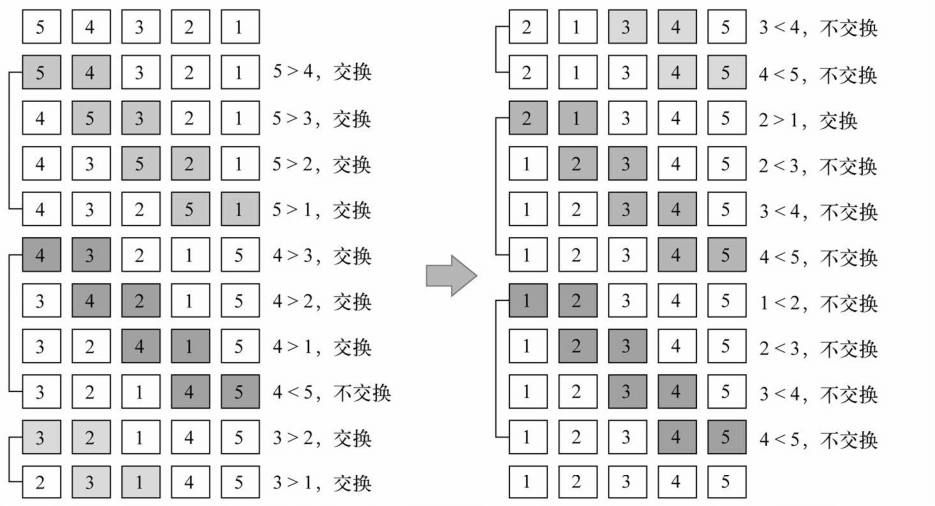function createNonSortedArray(size){
var array = new ArrayList();
for(var i = size; i > 0; i--){
array.insert(i);
}
return array;
}

var array = createNonSortedArray(5);
console.log(array.toString()); // 5,4,3,2,1
array.bubbleSort();
console.log(array.toString());	// 1,2,3,4,5


[5,4,3,2,1]

// 改进后的冒泡排序
this.modifiedBubbleSort = function(){
var length = array.length;
for(var i = 0; i <length; i++){
for(var j = 0; j < length-1-i; j++){
if(array[j] > array[j+1]){
this.swap(j,j+1);
}
}
}
}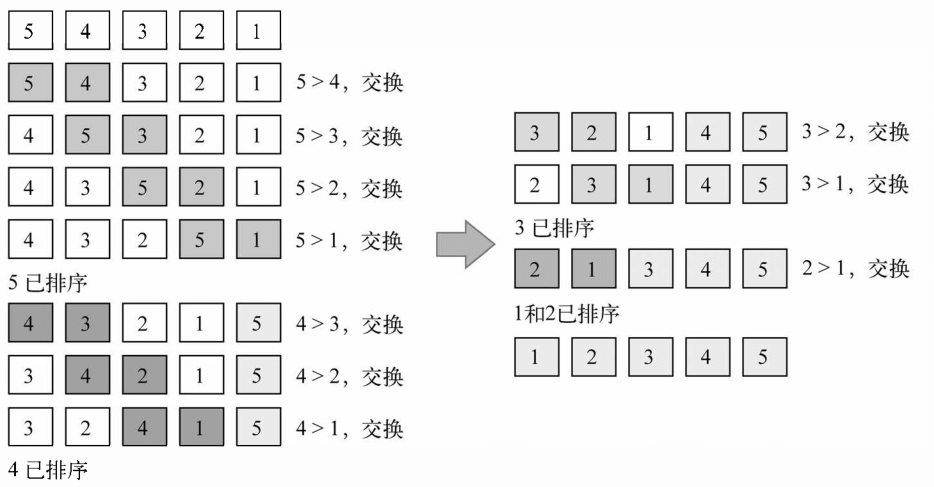### 选择排序

// 选择排序
this.selectionSort = function(){
var length = array.length,
indexMin;
for(var i = 0; i < length; i++){
indexMin = i;
for(var j = i; j < length; j++){
if(array[indexMin] > array[j]){
indexMin = j;
}
}
if(i !== indexMin){
this.swap(i,indexMin);
}
}
}


var array = createNonSortedArray(5);
console.log(array.toString()); // 1,2,3,4,5
array.selectionSort();
console.log(array.toString());	// 1,2,3,4,5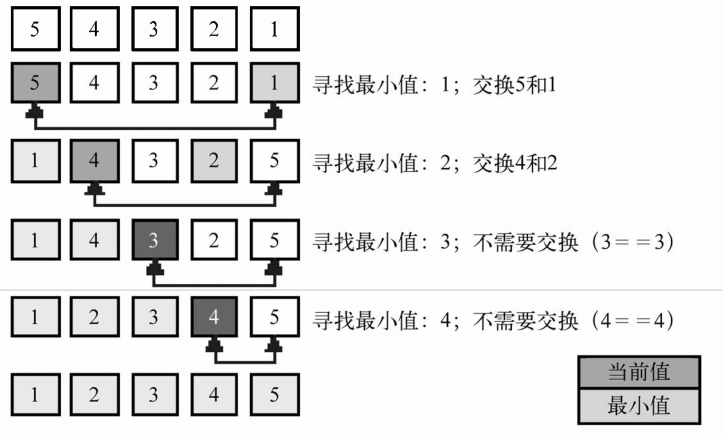### 插入排序

// 插入排序
this.insertionSort = function(){
var length = array.length,
j,
temp;
for(var i = 0; i <length; i++){
j = i;
temp = array[i];
while(j > 0 && array[j - 1] > temp){
array[j] = array[j - 1]
j--;
}
array[j] = temp;
}
}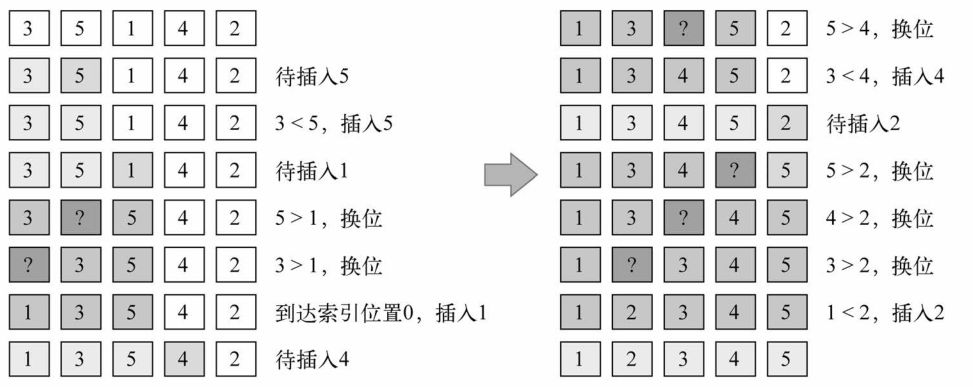### 归并排序

// 归并排序
this.mergeSort = function(){
array = this.mergeSortRec(array);
}


mergeSortRec 是递归函数

this.mergeSortRec = function(array){
var length = array.length;
if(length === 1){
return array;
}
var mid = Math.floor(length/2),
left = array.slice(0,mid),
right = array.slice(mid,length);
return merge(arguments.callee(left),arguments.callee(right));
}


function merge(left,right){
var result = [],
il = 0,
ir = 0;
while(il < left.length && ir < right.length){
if(left[il] < right[ir]){
result.push(left[il++]);
}else{
result.push(right[ir++]);
}
}
while(il < left.length){
result.push(left[il++]);
}
while(ir < right.length){
result.push(right[ir++]);
}
return result;
}


merge 函数接受两个数组作为参数，并将它们归并至一个大数组。排序发生在归并过程中。首先，需要声明归并过程要创建的新数组已经用来迭代两个数组（left和right数组）所需要的两个变量。迭代两个数组的过程中，我们来自left 数组的项是否比来自right的数组的项小。如果是，将该项从left数组添加至归并结果数组，并递增迭代数组的控制变量；否则，从right数组添加项并递增相应的迭代数组的控制变量。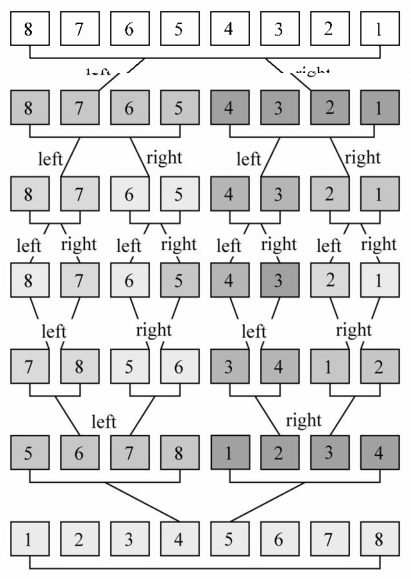### 快速排序

1. 首先，从数组中选择中间一项作为主元
2. 创建两个指针，左边一个指向数组的第一项，右边一个指向数组最后一项。移动左指针知道我们找到一个比主元大的元素，接着，移动右指针直到找到一个比主元小的元素，然后交换它们，重复这个过程，直到左指针超过了右指针。这个过程将使得比主元小的值都排在主元之前，而比主元大的值都排在主元之后。这一步叫做划分操作。
3. 接着，算法对划分后的小数组（较主元小的值组成的子数组，以及较主元大的值组成的子数组）重复之前的两个步骤，直到数组已完全排序。

// 快速排序
this.quickSort = function(){
this.quick(array,0,array.length-1);
}


this.quick = function(array,left,right){
var index;
if(array.length > 1){
index = partition(array,left,right);
if(left < index - 1 ){
arguments.callee(array,left,index-1);
}
if(index < right){
arguments.callee(array,index,right);
}
}
}


function partition(array,left,right){
var pivot = array[Math.floor((left+right) / 2)],
i = left,
j = right;
while(i <= j){
while(array[i] < pivot){
i++
}
while(array[j] > pivot){
j--
}
if(i <= j){
[array[i],array[j]] = [array[j],array[i]];
i++;
j--
}
}
return i;
}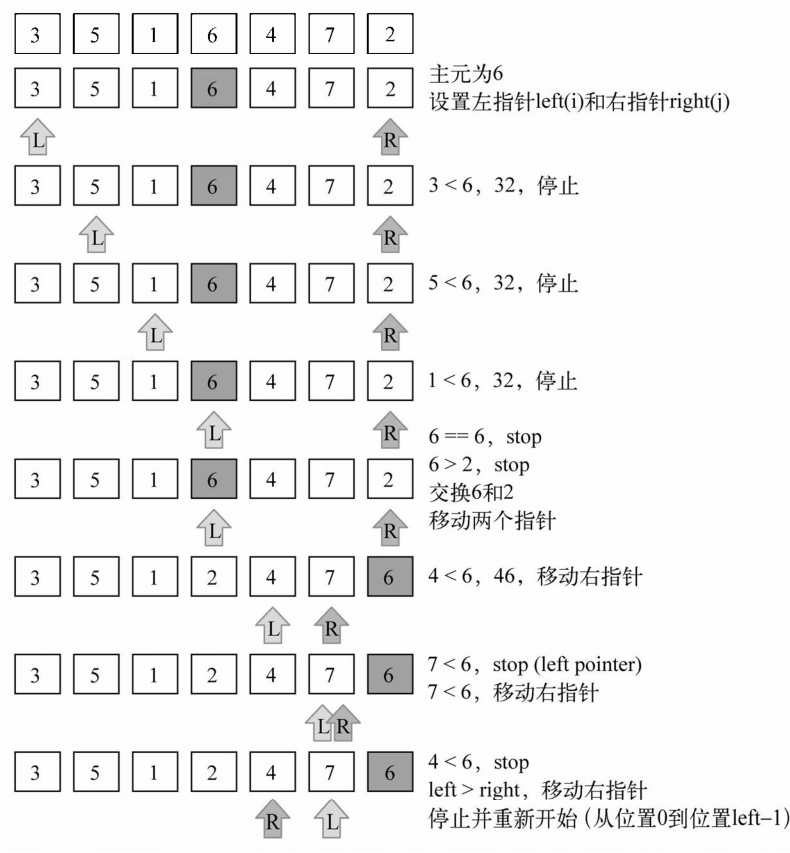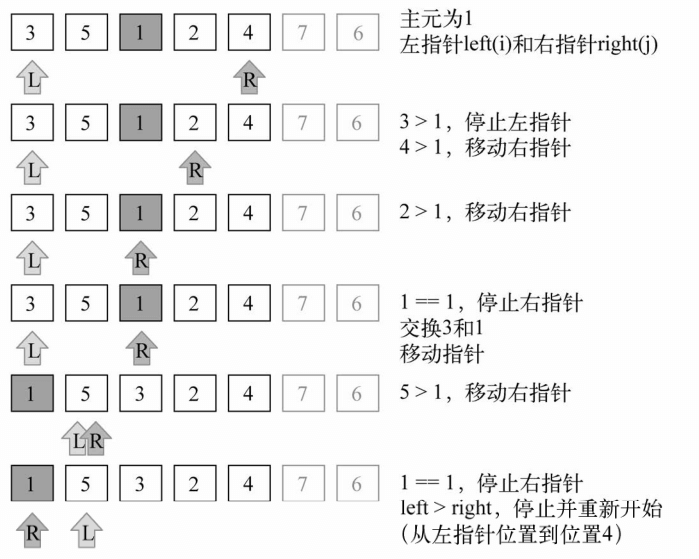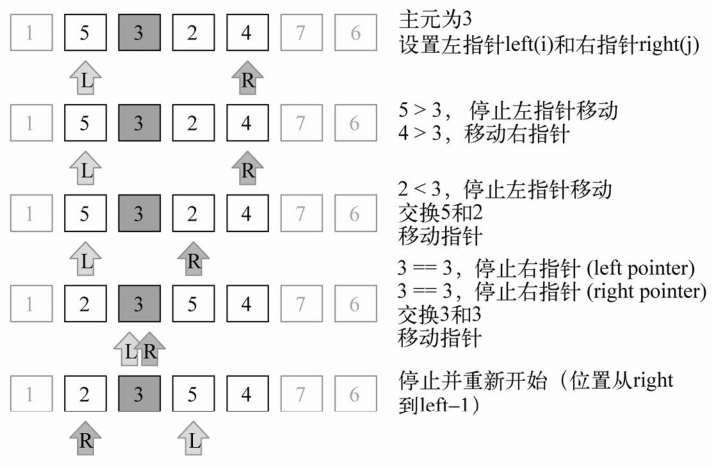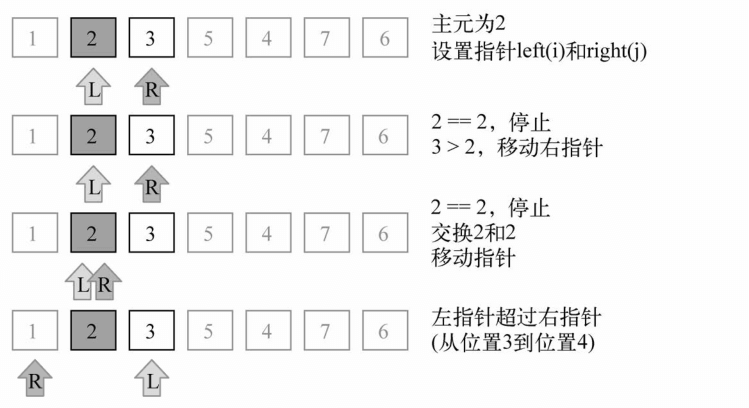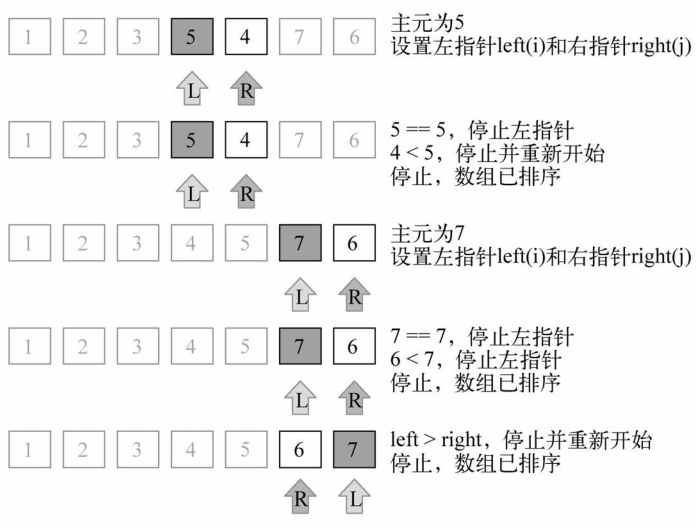### 排序的相关代码

function createNonSortedArray(size){
var array = new ArrayList();
for(var i = size; i > 0; i--){
array.insert(i);
}
return array;
}
function merge(left,right){
var result = [],
il = 0,
ir = 0;
while(il < left.length && ir < right.length){
if(left[il] < right[ir]){
result.push(left[il++]);
}else{
result.push(right[ir++]);
}
}
while(il < left.length){
result.push(left[il++]);
}
while(ir < right.length){
result.push(right[ir++]);
}
return result;
}
function partition(array,left,right){
var pivot = array[Math.floor((left+right) / 2)],
i = left,
j = right;
while(i <= j){
while(array[i] < pivot){
i++
}
while(array[j] > pivot){
j--
}
if(i <= j){
[array[i],array[j]] = [array[j],array[i]];
i++;
j--
}
}
return i;
}
function ArrayList(){
var array = [];
this.insert = function(item){
array.push(item);
}
this.toString = function(){
return array.join();
}
this.swap = function(index1,index2){
var aux = array[index1];
array[index1] = array[index2];
array[index2] = aux;
// [array[index1],array[index2]] = [array[index2],array[index1]]
}
// 冒泡排序
this.bubbleSort = function(){
var length = array.length;
for(var i = 0; i <length; i++){
for(var j = 0; j < length -1; j++){
if(array[j] > array[j+1]){
this.swap(j,j+1);
}
}
}
}
// 改进后的冒泡排序
this.modifiedBubbleSort = function(){
var length = array.length;
for(var i = 0; i <length; i++){
for(var j = 0; j < length-1-i; j++){
if(array[j] > array[j+1]){
this.swap(j,j+1);
}
}
}
}
// 选择排序
this.selectionSort = function(){
var length = array.length,
indexMin;
for(var i = 0; i < length; i++){
indexMin = i;
for(var j = i; j < length; j++){
if(array[indexMin] > array[j]){
indexMin = j;
}
}
if(i !== indexMin){
this.swap(i,indexMin);
}
}
}
// 插入排序
this.insertionSort = function(){
var length = array.length,
j,
temp;
for(var i = 0; i <length; i++){
j = i;
temp = array[i];
while(j > 0 && array[j - 1] > temp){
array[j] = array[j - 1]
j--;
}
array[j] = temp;
}
}
// 归并排序
this.mergeSort = function(){
array = this.mergeSortRec(array);
}
this.mergeSortRec = function(array){
var length = array.length;
if(length === 1){
return array;
}
var mid = Math.floor(length/2),
left = array.slice(0,mid),
right = array.slice(mid,length);
return merge(arguments.callee(left),arguments.callee(right));
}
// 快速排序
this.quickSort = function(){
this.quick(array,0,array.length-1);
}
this.quick = function(array,left,right){
console.log(left+'  '+right);
var index;
if(array.length > 1){
index = partition(array,left,right);
if(left < index - 1 ){
arguments.callee(array,left,index-1);
}
if(index < right){
arguments.callee(array,index,right);
}
}
}
}

// var array = createNonSortedArray(5);
// console.log(array.toString());
// array.quickSort();
// console.log(array.toString());
const quickSortArray = new ArrayList();
quickSortArray.insert(3);
quickSortArray.insert(5);
quickSortArray.insert(1);
quickSortArray.insert(6);
quickSortArray.insert(4);
quickSortArray.insert(7);
quickSortArray.insert(2);
console.log(quickSortArray.toString());
quickSortArray.quickSort();
console.log(quickSortArray.toString());


### 顺序搜索

// 顺序搜索
this.sequentialSearch = function(item){
for(var i = 0; i < array.length; i++){
if(item === array[i]){
return i;
}
}
return -1;
}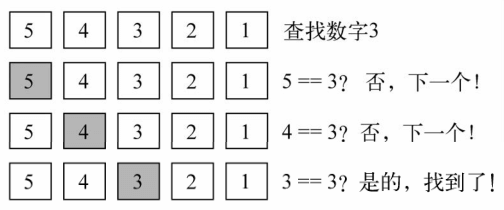### 二分搜索

1. 选择数组的中间值
2. 如果选中值是待搜索值，那么算法执行完毕
3. 如果待搜索值比选中的小，则返回步骤1并在选中值的左边的子数组中寻找
4. 如果待搜索值比选中的大，则返回步骤1并在选中值的右边的子数组中寻找

// 二分搜索
this.binarySearch = function(item){
this.quickSort();
var low = 0,
high = array.length - 1,
mid,element;
while(low <= high){
mid = Math.floor((low + high)/2);
element = array[mid];
if(element < item){
low = mid + 1;
}else if(element > item){
high = mid - 1;
}else{
return mid;
}
}
return -1;
}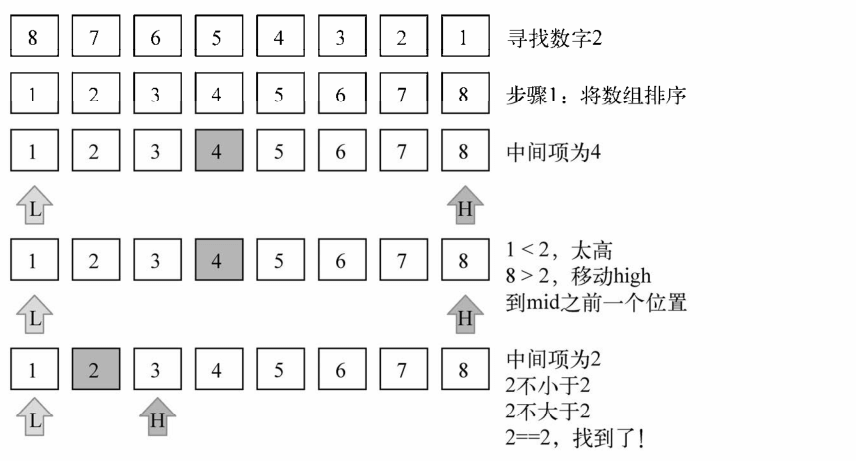### 小结

posted @ 2018-11-17 08:23  赖同学  阅读(625)  评论(0编辑  收藏  举报